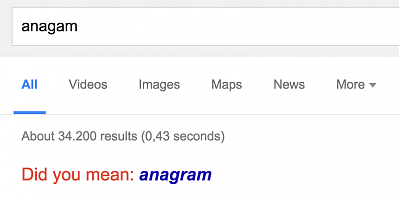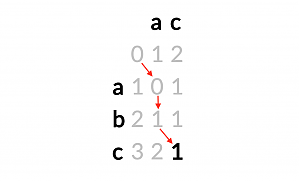# kleemans.ch

## Levenshtein distance

May 30, 2016

The Levenshtein distance or edit distance is a measure of how similar two strings are. For example, if we have two words like foot and tooth, the edit distance is exactly 2: first we replace the "f" with a "t", and then we add a "h" at the end:

• foot -> toot | f->t
• toot -> tooth | +h

A common application are spelling checkers or search engines. If for example you're searching on google and you enter a word with a typo, it's most likely recognized by the engine and a suggestion is given:Google will search for correctly written words with a small edit distance.

The Levenshtein distance is one way to measure how we get from one string to another. The following operations are allowed and also weighted the same:

• insertion
• substitution
• deletion

For each insertion, substitution or deletion, +1 is added to the edit distance.

## Demo 1 - Fruit search

Try searching a fruit from the following list: [apple, banana, pear, lemon]

Word:

Did you mean: {{best_match}}? (edit distance of {{best_val}})

For example, try typing "lemon" in the box below, you'll notice after "le..." that the word apple is shown (because it also has the part "le" in it), but after "lem", the suggestion will switch to lemon, because the edit distance is smaller.

## Wagner-Fischer algorithm

The calculation of the distance is not as trivial as it seems on first sight: For example if we take apple and lemon, how to start? After some trying around we discover that we can keep the "le"-part from apple, delete the rest and add mon to get lemon:

• apple -> pple -> ple -> le -> lem -> lemo -> lemon (6 steps)

But then we see that we can replace every single character of apple to get to lemon, and this will use only 5 operations, so the edit distance is really 5, not 6. How can we be sure to find the shortest way all the time?

Luckily, the calculation can be done with a matrix which keeps track of the optimal distance using the Wagner-Fischer algorithm. We start at the left upper corner and work our way down to the bottom right, with inserting, substituting and deleting:We want to get from abc to ac and start at 0. We see that "a" equals "a" and take the diagonal step to the next 0, just taking the exact same first character "a". Then we could either replace the "b" with a "c" (that would be the diagonal step and is also calculated), but instead we just delete a "b" and go down, because now we can make once again a diagonal step, taking the left over "c" at no cost. We end up at 1, which is our final (optimal) edit distance.

This is just a very quick demonstration, the algorithm works its way trough every cell of the matrix, in a Dynamic Programming kind-of way.

Have a look at the next demo:

## Demo 2 - full matrix

Here's a full matrix view of what happens when calculating the Levenshtein distance. Just edit the words and the matrix will change accordingly:

Word 1:

Word 2:

Levenshtein-Distance: {{matrix[word1.length][word2.length]}}

 {{letter}} {{(" " + word1).substring(\$index, \$index+1)}} {{item}}{{item}}

## Code

The following is a (shortened) implementation in Javascript:

``````// initialization of first row/column
for (var i = 0; i <= s.length; ++i) d[i] = i;
for (var i = 0; i <= t.length; ++i) d[i] = i;
// loop through matrix cells
for (var i = 1; i <= s.length; ++i) {
for (var j = 1; j <= t.length; ++j) {
if (s[i-1] == t[j-1]) { cost = 0; }
else { cost = 1; }
d[i][j] = Math.min(d[i-1][j]+1,
Math.min(d[i][j-1]+1,d[i-1][j-1]+cost));
}
}``````

Full code is also on Github.